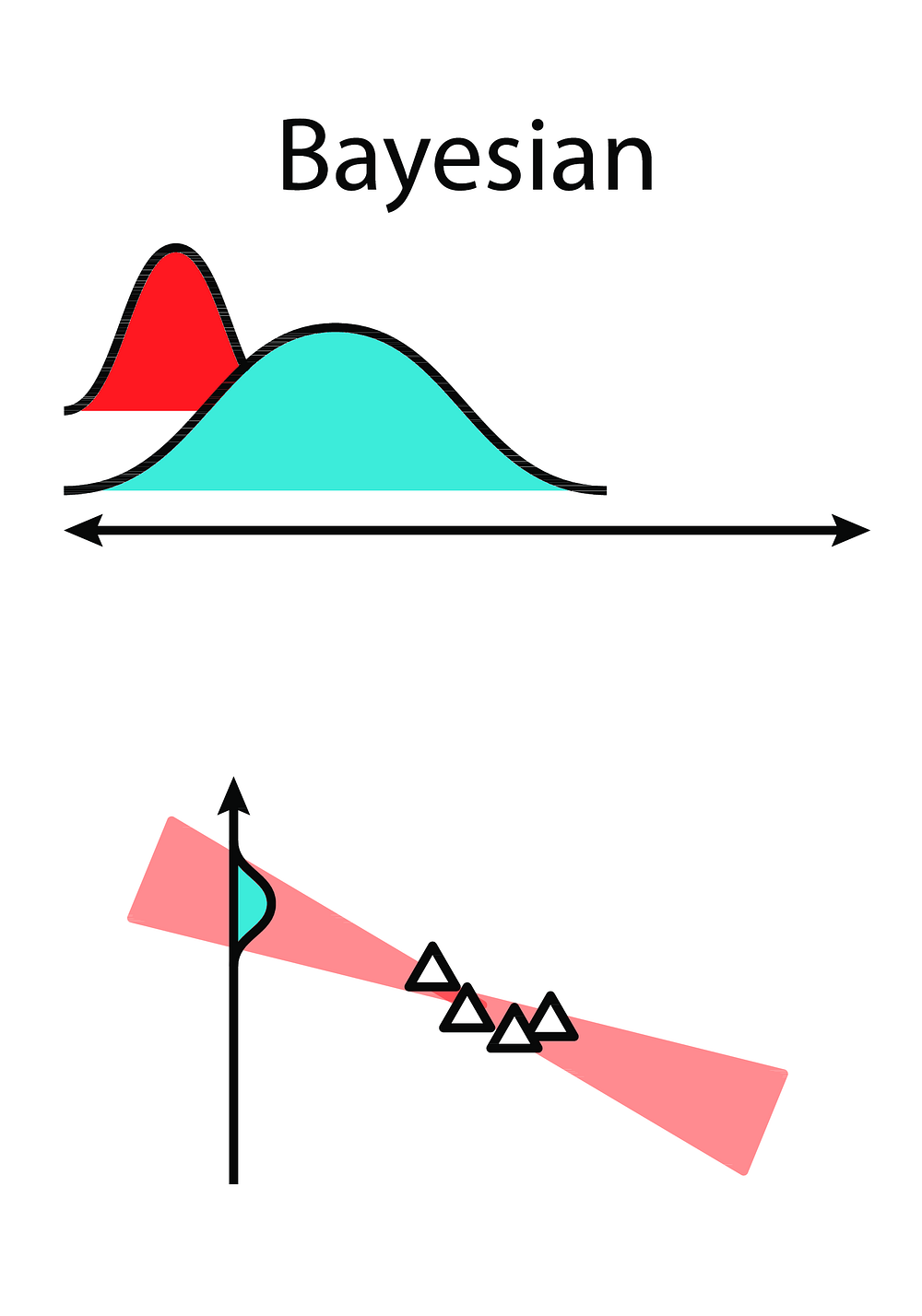# BLiTZ — A Bayesian Neural Network library for PyTorch

Original article can be found here (source): Deep Learning on Medium# BLiTZ — A Bayesian Neural Network library for PyTorch

## Blitz — Bayesian Layers in Torch Zoo is a simple and extensible library to create Bayesian Neural Network layers on the top of PyTorch.

This is a post on the usage of a library for Deep Bayesian Learning. If you are new to the theme, you may want to seek one of the many posts on medium about it or just the documentation section on Bayesian DL of our lib repo.

As there is a rising need for gathering uncertainty over neural network predictions, using Bayesian Neural Network layers became one of the most intuitive approaches — and that can be confirmed by the trend of Bayesian Networks as a study field on Deep Learning.

It occurs that, despite the trend of PyTorch as a main Deep Learning framework (for research, at least), no library lets the user introduce Bayesian Neural Network layers intro their models with as ease as they can do it with `nn.Linear` and `nn.Conv2d`, for example.

Logically, that causes a bottleneck for anyone that wants to iterate flexibly with Bayesian approaches for their data modeling, as the user has to develop the whole part of Bayesian Layers for its use rather than focusing on the architecture of its model.

BLiTZ was created to change to solve this bottleneck. By being fully integrated with PyTorch (including with `nn.Sequential` modules) and easy to extend as a Bayesian Deep Learning library, BLiTZ lets the user introduce uncertainty on its neural networks with no more effort than tuning its hyper-parameters.

In this post, we discuss how to create, train and infer over uncertainty-introduced Neural Networks, using BLiTZ layers and sampling utilities.

# Bayesian Deep Learning layers

As we know, the main idea on Bayesian Deep Learning is that, rather than having deterministic weights, at each feed-forward operation, the Bayesian layers samples its weights from a normal distribution.

Consequently, the trainable parameters of the layer are the ones that determine the mean and variance of this distribution.

Mathematically, the operations would go from:

To: